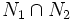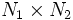# Equivalence of internal and external direct product

This article gives a proof/explanation of the equivalence of multiple definitions for the term direct product
View a complete list of pages giving proofs of equivalence of definitions

## The definitions we have to prove as equivalent

### Internal direct product

Suppose$G$ is a group, and$N_1, N_2$ are normal subgroups. We say that$G$ is an internal direct product of$N_1$ and$N_2$ if$N_1 \cap N_2$ is trivial, and$N_1N_2 = G$.

## What we need to prove

### Every internal direct product is naturally isomorphic to the external direct product

We need to prove that given a group$G$ that is the internal direct product of normal subgroups$N_1, N_2$, we can identify$G$ naturally with the external direct product$N_1 \times N_2$.

Proof: Consider the map:$\alpha: N_1 \times N_2 \to G, \qquad \alpha(a,b) = ab$

We first must verify that$\alpha$ is a group homomorphism. For this, we first observe that if$a \in N_1, b \in N_2$, then:$aba^{-1}b^{-1} = a(ba^{-1}b^{-1}) \in N_1; \qquad aba^{-1}b^{-1} = (aba^{-1})b^{-1} \in N_2$

Combining these, we get:$aba^{-1}b^{-1} \in N_1 \cap N_2 = \{ e \}$

Hence, we get:$ab = ba$.

Thus, any element of$N_1$ commutes with any element of$N_2$. Thus, if we pick two pairs$(a,b), (c,d) \in N_1 \times N_2$, then:$\alpha(a,b)\alpha(c,d) = abcd = a(bc)d = a(cb)d = (ac)(bd) = \alpha(ac,bd) = \alpha((a,b)(c,d))$

In the intermediate step, we use that$b$ and$c$ commute. Thus,$\alpha$ is a group homomorphism. It's clear that$\alpha$ preserves the identity element and inverses, too.

Next we note that:

1.$\alpha$ is injective because$\alpha(a,b) = e \implies ab = e \implies b = a^{-1} \implies a,b \in N_1 \cap N_2 = \{ e \}$.
2.$\alpha$ is surjective because by assumption,$N_1N_2 = G$.

Thus,$\alpha$ defines an isomorphism from$N_1 \times N_2$ to$G$.

### Every external direct product is naturally realized as an internal direct product

We need to show that if$A$ and$B$ are abstract groups, then we can find subgroups in$A \times B$ isomorphic to$A$ and$B$ respectively, whose internal direct product is$A \times B$. Indeed:

1. The subgroup isomorphic to$A$ is$A \times \{ e \}$ (i.e., elements of the form$(a,e)$).
2. The subgroup isomorphic to$B$ is$\{ e \} \times B$ (i.e., elements of the form$(e,b)$).

Let's now check the conditions for an internal direct product:

1. Both the subgroups are normal: This is a direct check using the coordinate-wise multiplication.
2. The subgroups intersect only at$(e,e)$: This is set-theoretically obvious.
3. The product of the subgroups is$A \times B$: Any element$(a,b)$ can be written as the product of$(a,e)$ and$(e,b)$.